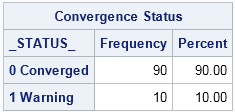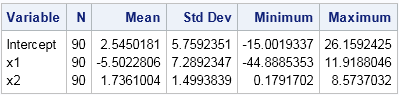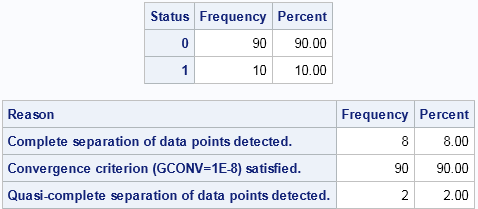Ugh! Your favorite regression procedure just printed a warning to the SAS log. Something is wrong, and your attempt to fit a model to the data has not succeeded. A typical message is "WARNING: The validity of the model fit is questionable," perhaps followed by some additional diagnostic messages about "quasi-separation" or "lack of convergence."

If your modeling toolkit includes procedures such as LOGISTIC, GENMOD, MIXED, NLMIXED, NLIN, or PHREG, you might have experienced convergence problems. A small sample size or a misspecified model are among the reasons for lack of convergence. There are many papers that discuss how to handle convergence issues. Paul Allison (2008) wrote a paper on some reasons that a logistic model might fail to converge, including an explanation of quasi-complete separation. The documentation for the MIXED procedure includes a long list of potential reasons that a mixed model might fail to converge. Several of my friends from SAS Technical Support give advice on convergence in their SAS Global Forum paper (Kiernan, Tao, and Gibbs, 2012).

Although it can be frustrating to deal with convergence issues during a data analysis, lack-of-convergence during a simulation study can be maddening. Recall that the efficient way to implement a simulation study in SAS is to use BY-group processing to analyze thousands of independent samples. A simulation study is designed to run in batch mode without any human intervention. How, then, can the programmer deal with lack of convergence during a simulation?

Some SAS users (such as Chen and Dong, 2009) have suggested parsing the SAS log for notes and warning messages, but that approach is cumbersome. Furthermore, if you have turned off SAS notes during the simulation, then there are no notes in the log to parse!

### The _STATUS_ variable in the OUTEST= data set

Fortunately, SAS procedures that perform nonlinear optimization provide diagnostic variables as part of their output. These variables are informally known as "status variables." You can monitor the status variables to determine the BY groups for which the optimization converged.

The easiest way to generate a status variable is to use the OUTEST= option to generate an output data set that contains parameter estimates. Not every procedure supports the OUTEST= option, but many do. Let's see how it works. In my article "Simulate many samples from a logistic regression model," I showed how to generate 100 samples of data that follow a logistic regression model. If you make the sample size very small (like N=20), PROC LOGISTIC will report convergence problems for some of the random samples, as follows:

```%let N = 20; /* N = sample size */ %let NumSamples = 100; /* number of samples */ /* Generate logistic data: See https://blogs.sas.com/content/iml/?p=11735 */   options nonotes; /* turn of notes; use OUTEST= option */ proc logistic data=LogisticData noprint outest=PE; by SampleId; model y(Event='1') = x1 x2; run; options notes;   proc freq data=PE; tables _STATUS_ / nocum; run;```The output from PROC FREQ shows that 10% of the models did not converge. The OUTEST= data set has a variable named _STATUS_ that indicates whether the logistic regression algorithm converged. You can use the _STATUS_ variable to analyze only those parameter estimates from converged optimizations. For example, the following call to PROC MEANS produces summary statistics for the three parameter estimates in the model, but only for the converged models:

```proc means data=PE nolabels; where _STATUS_ = "0 Converged" /* analyze estimates for converged models */ var Intercept x1 x2; run;```### The ConvergenceStatus table

Notice that the _STATUS_ variable does not contain details about why the algorithm failed to converge. For that information, you can use the ConvergenceStatus ODS table, which is produced by all SAS/STAT regression procedures that involve nonlinear optimization. To save the ODS table to an output data set, you cannot use the NOPRINT option. Instead, use the %ODSOff and %ODSOn macros to suppress ODS output. Use the ODS OUTPUT statement to write the ConvergenceStatus tables to a SAS data set, as follows:

```/* define %ODSOff and %ODSOn macros */ %ODSOff proc logistic data=LogisticData; by SampleId; model y(Event='1') = x1 x2; ods output ParameterEstimates=PE2 ConvergenceStatus=CS; run; %ODSOn   proc freq data=CS; tables Status Reason / nocum; run;```The ConvergenceStatus table has a numerical variable named Status, which has the value 0 if the algorithm converged. The character variable Reason contains a short description of the reason that the algorithm terminated. The results of the PROC FREQ call shows that the logistic algorithm failed eight times because of "complete separation," converged 90 times, and failed twice due to "quasi-complete separation."

You can use the DATA step and the MERGE statement to merge the ConvergenceStatus information with the results of other ODS tables from the same analysis. For example, the following statements merge the convergence information with the ParameterEstimates table. PROC MEANS can be used to analyze the parameter estimates for the models that converged. The summary statistics are identical to the previous results and are not shown.

```data All; merge PE2 CS; by SampleID; run;   proc means data=All; where Status = 0; class Variable; var Estimate; run;```

In conclusion, the ConvergenceStatus table provides information about the convergence for each BY group analysis. When used as part of a simulation study, you can use the ConvergenceStatus table to manage the analysis of the simulated data. You can count the number of samples that did not converge, you can tabulate the reasons for nonconvergence, and you can exclude nonconverged estimates from your Monte Carlo summaries.

ShareDistinguished Researcher in Computational Statistics

Rick Wicklin, PhD, is a distinguished researcher in computational statistics at SAS and is a principal developer of SAS/IML software. His areas of expertise include computational statistics, simulation, statistical graphics, and modern methods in statistical data analysis. Rick is author of the books Statistical Programming with SAS/IML Software and Simulating Data with SAS.

1.••